# The MI Procedure

### Monotone and FCS Logistic Regression Methods

Subsections:

The logistic regression method is another imputation method available for classification variables. In the logistic regression method, a logistic regression model is fitted for a classification variable with a set of covariates constructed from the effects, where the classification variable is an ordinal response or a nominal response variable.

In the MI procedure, ordered values are assigned to response levels in ascending sorted order. If the response variable Y takes values in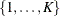, then for ordinal response models, the cumulative model has the form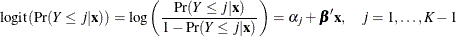where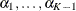are K-1 intercept parameters, and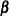is the vector of slope parameters.

For nominal response logistic models, where the K possible responses have no natural ordering, the generalized logit model has the form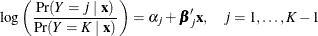where theare K-1 intercept parameters, and the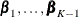are K-1 vectors of slope parameters.

#### Binary Response Logistic Regression

For a binary classification variable, based on the fitted regression model, a new logistic regression model is simulated from the posterior predictive distribution of the parameters and is used to impute the missing values for each variable (Rubin, 1987, pp. 167–170).

For a binary variable Y with responses 1 and 2, a logistic regression model is fitted using observations with observed values for the imputed variable Y: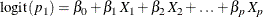where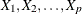are covariates for Y,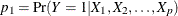,   andThe fitted model includes the regression parameter estimates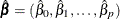and the associated covariance matrix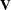.

The following steps are used to generate imputed values for a binary variable Y with responses 1 and 2:

1. New parameters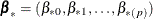are drawn from the posterior predictive distribution of the parameters.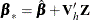where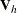is the upper triangular matrix in the Cholesky decomposition,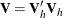, andis a vector of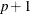independent random normal variates.

2. For an observation with missing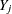and covariates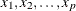, compute the predicted probability that Y= 1: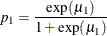where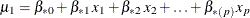.

3. Draw a random uniform variate, u, between 0 and 1. If the value of u is less than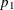, impute Y= 1; otherwise impute Y= 2.

The binary logistic regression imputation method can be extended to include the ordinal classification variables with more than two levels of responses, and the nominal classification variables. The LINK=LOGIT and LINK=GLOGIT options can be used to specify the cumulative logit model and the generalized logit model, respectively. The options ORDER= and DESCENDING can be used to specify the sort order for the levels of the imputed variables.

#### Ordinal Response Logistic Regression

For an ordinal classification variable, based on the fitted regression model, a new logistic regression model is simulated from the posterior predictive distribution of the parameters and is used to impute the missing values for each variable.

For a variable Y with ordinal responses 1, 2, …, K, a logistic regression model is fitted using observations with observed values for the imputed variable Y: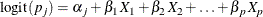whereare covariates for Y and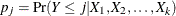.

The fitted model includes the regression parameter estimates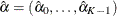and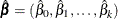, and their associated covariance matrix.

The following steps are used to generate imputed values for an ordinal classification variable Y with responses 1, 2, …, K:

1. New parameters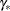are drawn from the posterior predictive distribution of the parameters.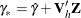where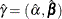,is the upper triangular matrix in the Cholesky decomposition,, andis a vector of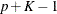independent random normal variates.

2. For an observation with missing Y and covariates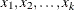, compute the predicted cumulative probability for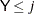: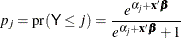3. Draw a random uniform variate, u, between 0 and 1, then impute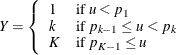#### Nominal Response Logistic Regression

For a nominal classification variable, based on the fitted regression model, a new logistic regression model is simulated from the posterior predictive distribution of the parameters and is used to impute the missing values for each variable.

For a variable Y with nominal responses 1, 2, …, K, a logistic regression model is fitted using observations with observed values for the imputed variable Y: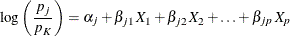whereare covariates for Y and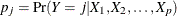.

The fitted model includes the regression parameter estimatesand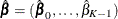, and their associated covariance matrix, where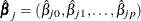,

The following steps are used to generate imputed values for a nominal classification variable Y with responses 1, 2, …, K:

1. New parametersare drawn from the posterior predictive distribution of the parameters.where,is the upper triangular matrix in the Cholesky decomposition,, andis a vector ofindependent random normal variates.

2. For an observation with missing Y and covariates, compute the predicted probability for Y= j, j=1, 2, …, K-1: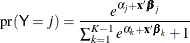and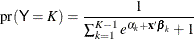3. Compute the cumulative probability for: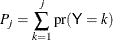4. Draw a random uniform variate, u, between 0 and 1, then impute